Practice the AP 7th Class Maths Bits with Answers 5th Lesson Triangles on a regular basis so that you can attempt exams with utmost confidence.

## AP Board 7th Class Maths Bits 5th Lesson Triangles

Multiple Choice Questions:

Question 1.
A. simple closed plane figure formed by three line segments is called ___________
(A) Angle
(B) Triangle
(C) Rectangle
(D) Square
(B) Triangle

Question 2.
Triangle has ___________
(A) 3 sides
(B) 3 angles
(C) 3 vertices
(D) all of the above
(D) all of the above

Question 3.
A triangle with two equal sides is called ___________ triangle.
(A) Isosceles
(B) Equilateral
(C) Scalene
(D) Obtuse angled
(A) Isosceles

Question 4.
A triangle with no two sides are equal is called ___________ triangle.
(A) Isosceles
(B) Equilateral
(C) Scalene
(D) Obtuse angled
(C) ScaleneQuestion 5.
A triangle with all acute angle is called ___________ triangle.
(A) Right angled
(B) Obtuse angled
(C) Acute angled
(D) Equilateral
(C) Acute angled

Question 6.
Sum of the interior angles of a triangle is ___________
(A) Two right angles
(B) One straight angle
(C) A & B
(D) None
(C) A & B

Question 7.
In a triangle sum of its any two interior angles is equal to ___________
(A) 90°
(B) 180°
(C) Interior angle
(D) Exterior angle
(D) Exterior angle

Question 8.
The sum of the lengths of any two sides of a triangle is ___________ the length of the third side.
(A) greater than
(B) less than
(C) equal to
(D) all of the above
(A) greater than

Question 9.
The difference of the lengths of any two sides of a triangle is ___________ the length of the third side.
(A) greater than
(B) less than
(C) equal to
(D) all of the above
(B) less than

Question 10.
In ∆PQR, PQ – QR ….. PR
(A) >
(B) <
(C) =
(D) ≠
(B) <

Question 11.
In any triangle the opposite side to the smallest angle is ___________ the other two sides.
(A) bigger than
(B) equal to
(C) smaller than
(D) None
(C) smaller than

Question 12.
In a triangle the perpendicular line segment from any vertex to it’s opposite side is called ___________
(A) side
(B) diagonal
(C) altitude
(D) hypotenuse
(C) altitudeQuestion 13.
A triangle can have atmost ___________ right angles.
(A) 1
(B) 2
(C) 3
(D) 0
(A) 1

Question 14.
Altitude of an obtuse angled triangle lies ___________the triangle.
(A) inside
(B) outside
(C) on the triangle
(D) all of the above
(B) outside

Question 15.
In ∆ADI, AD = AI and ∠D = 45°, then the triangle is ___________
(A) Isosceles and acute angled
(B) Isosceles and obtuse angled
(C) Equilateral
(D) Isosceles and right angled
(D) Isosceles and right angled

Question 16.
One of the exterior angles of a triangle . is 120° and the interior opposite angles are in the ratio 2 : 3, then the angles are ___________
(A) 72°, 48°, 60°
(B) 70°, 50°, 60°
(C) 73°, 47°, 60°
(D) 75°, 40°,’ 65°
(A) 72°, 48°, 60°

Question 17.
In the figure x°, y° are =___________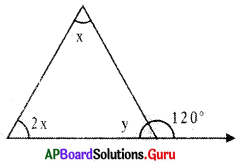(A) greater than
(B) less than
(C) equal to
(D) all of the above
(A) greater than

Question 18.
In the figure a° are = ___________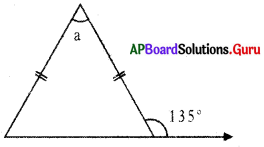(A) 45°
(B) 55°
(C) 90°
(D) 35°
(C) 90°

Question 19.
From the given figure ∠b = ___________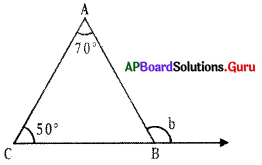(A) 130°
(B) 60°
(C) 20°
(D) 120°
(D) 120°

Question 20.
From the given figure ∠x – ∠y = ___________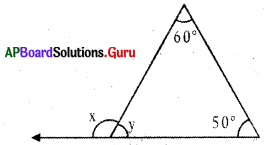(A) 70°
(B) 20°
(C) 40°
(D) 50°
(C) 40°Question 21.
In a right angled triangle one is 43°, then the third angle is ___________
(A) 45°
(B) 47°
(C) 57°
(D) 37°
(B) 47°

Question 22.
In ∆PQR, PQ = PR, then ∠Q = ___________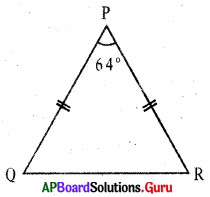(A) 45°
(B) 47°
(C) 57°
(D) 37°
(A) 45°

Question 23.
Which of the following measurements form a triangle ?
(A) 4 cm, 4 cm, 8 cm
(B) 5 cm, 6 cm, 11 cm
(C) 7 cm, 11 cm, 4 cm
(D) 7 cm, 8 cm, 9 cm
(D) 7 cm, 8 cm, 9 cm

Question 24.
Two angles of a triangle are 75° and 35°, then the measure of third angle is
(A) 35°
(B) 45°
(C) 70°
(D) 75°
(C) 70°

Question 25.
In the adjacent figure, if ED ∥ AB, ∠A = 60° and ∠B = 80°, then ∠BCD = ___________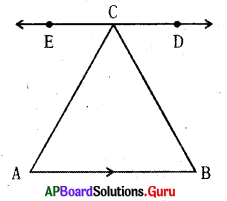(A) 140°
(B) 80°
(C) 60°
(D) 20°
(B) 80°

Fill in the blanks :

Question 1.
A triangle divides the plane into ___________ parts.
3

Question 2.
Triangle has ___________ vertices.
3Question 3.
If all the sides of a triangle are equal, then it is called ___________
Equilateral Triangle

Question 4.
A triangle with one right angle is called ___________
Right angled triangle

Question 5.
A triangle with one obtuse angle is called a ___________
Obtuse angled triangle

Question 6.
According to the measure of angles, triangles are classified into ___________
3 types

Question 7.
Sum of the interior angles of a triangle is ___________
180°

Question 8.
An exterior angle of a triangle is equal to the sum of its ___________
Opposite interior angles

Question 9.
In ∆ABC, AB + BC ___________ AC.
>

Question 10.
In any triangle the opposite side to the biggest angle is ___________ the other two sides.
biggger than

Question 11.
The sides which are opposite to equal angles are ___________
also equal

Question 12.
Exterior angle of an equilateral triangle is ___________
120°

Question 13.
Altitude of a right angled triangle lies ___________ the triangle.
inside

Question 14.
Sum of the exterior angles of triangle is ___________
360°

Question 15.
If one acute angle of a right angled triangle is 20°, the other angle is ___________
90°

Question 16.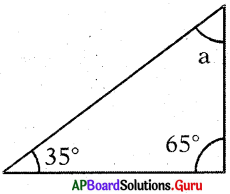From the given figure ∠a = ___________
80°

Question 17.
If the measure of two angles of a triangle are 35°, 84°, then the third angle is ___________
61°

Question 18.
Three angles of a triangle are (x + 30°), 2x, (3x – 30°), then x = ___________
30°

Question 19.
A triangle can have ___________ right angles.
1Question 20.
A triangle can have ___________ obtuse angles.
1

Question 21.
In an isosceles triangle the non-equal angle is 40°, then equal angles are ___________
70°, 70°

Question 22.
The angles of triangle are in the ratio 2:3:4, then the largest angle is ___________
80°

Question 23.
In ∆PQR, PQ = PR; ∠P – 50°, so, ∠Q = ___________
65°

Question 24.
The angles of a triangle are x, 2x and 3x + 60°, then x = ___________
20°

Question 25.
The side which is opposite to right . angle in the right angled triangle is called ___________
Hypotenuse

Match the following:

Question 1.

 Group A Group B 1. Equilateral triangle (A) all are acute angles 2. Isosceles triangle (B) one angle is 90° 3. Scalene triangle (C) all sides are equal 4. Acute angled triangle (D) one angle is obtuse 5. Obtuse angled triangle (E) two sides are equal 6. Right-angled triangle (F) sides are different in length